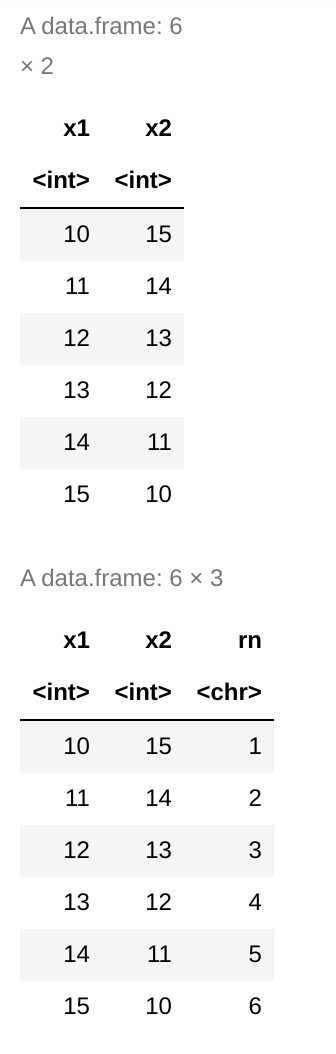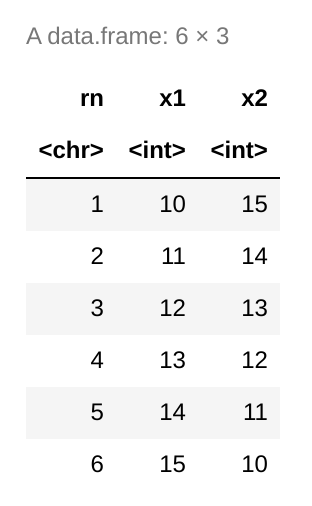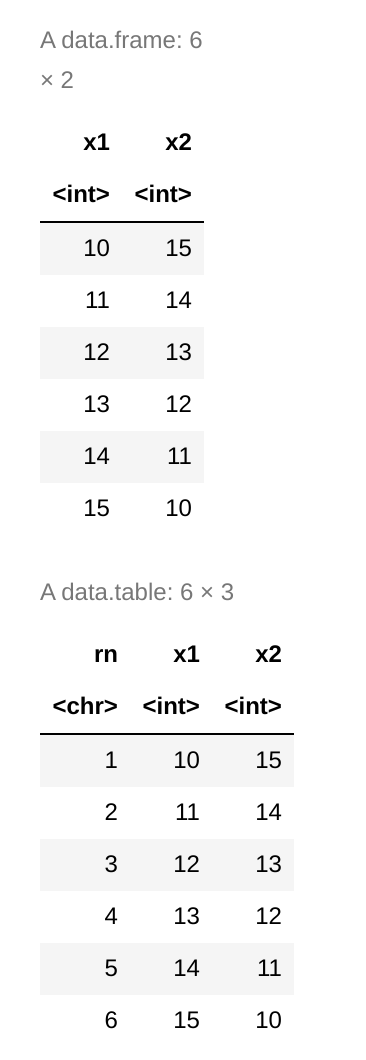Open in App
Not now

# Convert Row Names into Column of DataFrame in R

• Last Updated : 17 Nov, 2021

In this article, we will discuss how to Convert Row Names into Columns of Dataframe in R Programming Language.

### Method 1: Using row.names()

row.name() function is used to set and get the name of the DataFrame. Apply the row.name() function to the copy of the DataFrame and a name to the column which contains the name of the column with the help of the \$ sign.

Syntax:

row.names(dataframe)

Example:

## R

 `# DataFrame is created with the ` `# help of data.frame function ` `data <- ``data.frame``(x1 = (10:15),   ` `                   ``x2 = (15:10))`   `# print the DataFrame` `data`   `# make copy of DataFrame` `copydata <- data       `   `# apply row.name function to get` `# the name of the row` `copydata\$rn <- ``row.names``(data)   `   `# print the result` `copydata`

Output:### Method 2: Using dplyr

In the R language there’s a package named dplyr which performs several DataFrame tasks. So, we are going to add a row name into a column of a DataFrame with the help of this package. At first, we are going to install and load the dplyr package. After loading the package we follow the same steps we followed in the first method but this time with the help of the function of dplyr library. The function used in this method is rownames_to_columns().

Syntax:

tibble::rownames_to_column(data_frame, column_name)

Example:

## R

 `# DataFrame is created with the ` `# help of data.frame function ` `data <- ``data.frame``(x1 = (10:15),   ` `                   ``x2 = (15:10))`   `# load the package` `library``(``"dplyr"``)`   `# make the copy of the data frame` `copydata <- data   `   `# Apply rownames_to_column on the copy of ` `# DataFrame and put name of function rn` `copydata <- tibble::``rownames_to_column``(copydata, ``"rn"``) `   `# print the copied DataFrame` `copydata`

Output:### Method 3: Using data.table

In the R language there’s a package named data.table which performs several DataFrame tasks. So, we are going to add a row name into a column of a DataFrame with the help of this package. At first, we are going to install and load the data.table package. After loading the package we follow the same steps we followed in the first method but this time with the help of the function of data.table library. The function used in this method is setDT().

Syntax:

setDT(x, keep.rownames=TRUE/FALSE, key=NULL, check.names=TRUE/FALSE)

While using this syntax we keep the row name true to get the name of the row.

Example:

## R

 `# DataFrame is created with the help` `# of data.frame function ` `data <- ``data.frame``(x1 = (10:15),   ` `                   ``x2 = (15:10))`   `# print the DataFrame` `data`   `# load the library` `library``(``"data.table"``)`   `# make copy of dataframe` `copydata <- data          `   `# Apply setDT function with rowname true ` `# to get the rowname` `copydata <- ``setDT``(data, keep.rownames = ``TRUE``)[]          `   `# print the data` `copydata  `

Output:My Personal Notes arrow_drop_up
Related Articles